## Strassen Formulas

The usual number of scalar operations (i.e., the total number of additions and multiplications) required to perform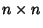Matrix Multiplication is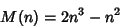(1)

(i.e.,multiplications andadditions). However, Strassen (1969) discovered how to multiply two Matrices in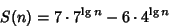(2)

scalar operations, whereis the Logarithm to base 2, which is less than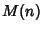for. Fora power of two (), the two parts of (2) can be written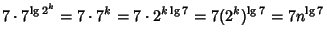(3)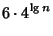(4)

so (2) becomes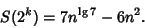(5)

Two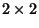matrices can therefore be multiplied(6)(7)

with only(8)

scalar operations (as it turns out, seven of them are multiplications and 18 are additions). Define the seven products (involving a total of 10 additions) as(9)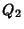(10)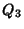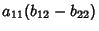(11)(12)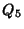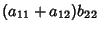(13)(14)(15)

Then the matrix product is given using the remaining eight additions as(16)(17)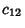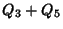(18)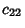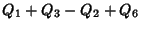(19)

(Strassen 1969, Press et al. 1989).

Matrix inversion of amatrixto yieldcan also be done in fewer operations than expected using the formulas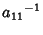(20)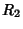(21)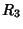(22)(23)(24)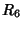(25)(26)(27)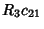(28)(29)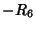(30)

(Strassen 1969, Press et al. 1989). The leading exponent for Strassen's algorithm for a Power of 2 is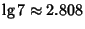. The best leading exponent currently known is 2.376 (Coppersmith and Winograd 1990). It has been shown that the exponent must be at least 2.

References

Coppersmith, D. and Winograd, S. Matrix Multiplication via Arithmetic Programming.'' J. Symb. Comput. 9, 251-280, 1990.

Pan, V. How to Multiply Matrices Faster. New York: Springer-Verlag, 1982.

Press, W. H.; Flannery, B. P.; Teukolsky, S. A.; and Vetterling, W. T. Is Matrix Inversion anProcess?'' §2.11 in Numerical Recipes in FORTRAN: The Art of Scientific Computing, 2nd ed. Cambridge, England: Cambridge University Press, pp. 95-98, 1989.

Strassen, V. Gaussian Elimination is Not Optimal.'' Numerische Mathematik 13, 354-356, 1969.